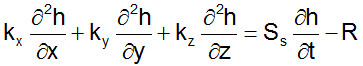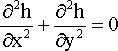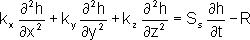# Fall Semester 2018

 Page 1 [Page 2] Page 3 Page 4 Page 5

Multiple Choice

(1 pt each)

2. (head-darcy) The units for hydraulic conductivity are:

 a. [L/T2] b. [L/T] c. [L2] d. [L2/T]

3. (goveq) Which of the following is not one of the basic assumptions we discussed when
deriving the governing 3D equations:

 a. The soil is isotropic b. Darcy's law is valid c. Mass is conserved d. Soil is saturated e. All of the above are basic assumptions

4. (gw-concepts) Adjusting the model parameters until the output matches field data at the site is called:

 a. post-audit b. verification c. calibration d. quality control

5. (goveq) A function representing a direct mathematical solution to the governing differential flow equation is called:

 a. A numerical solution. b. An analytical solution. c. A flow net. d. A flow field. e. A potentiometric surface. f. None of the above.

6. (modflow) Which of the following packages is always required by MODFLOW?

 a. Recharge b. Well c. General Head d. River e. Drain f. All of the above are optional

7. (modflow) Which of the following parameters is not required by the Drain package for each
drain cell?

 a. Drain elevation b. Conductance c. Flow rate d. All are required

8. (head-darcy) The off-diagonal terms of the 2D and 3D hydraulic conductivity tensor are nonzero when:

 a. The soil is homogenous b. The soil is isotropic c. The x and y axes are aligned with the principal axes of hydraulic conductivity d. None of the above

9. (head-darcy) Which of the following soils would have the largest difference between the Darcian velocity and the seepage velocity?

 a. Sands b. Gravels c. Fractured Rock d. All of the above are about the same.

10. (goveq) For the governing equation:which of the following conditions apply?

 a. isotropic b. anisotropic c. xyz axes aligned with principal axes of hyd. conductivity d. 2D e. 3D f. steady state

11. (goveq) For the following governing equation:which of the following conditions apply? Check all that apply.

 a. isotropic b. steady state c. 3D d. sources and sinks e. transient

12. (goveq) For the following governing equation:which of the following conditions apply?:

 a. 2D b. isotropic c. The x, y, and z axes correspond to the principal axes of hydraulic conductivity d. steady state only e. internal sources and sinks f. transient